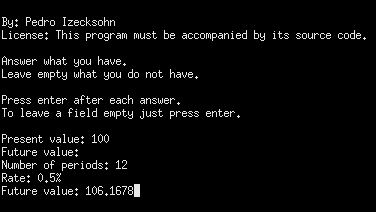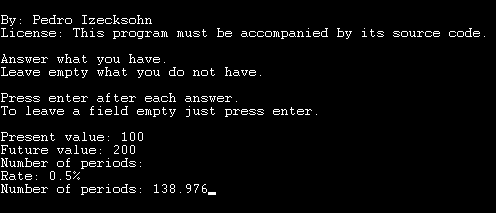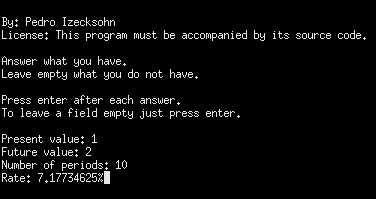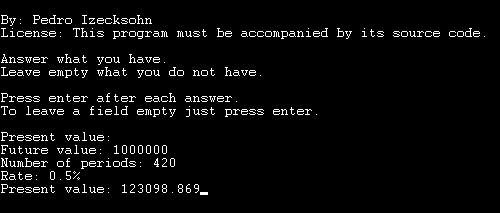rate
To calculate compound interest rate.

To calculate fixed rate loan or investment where the principal and the interest are paid at once on the end.

Author: Pedro Izecksohn
License: This program is distributable only if its sources and its author`s name be included in the package.

 It asks from you 4 questions, but you must answer only 3 of them. It will calculate for you the value that you leave it blank.

 The four questions are: present value, future value,number of periods,rate

Present value - is the value that you get on a loan or that you invest.

Future value - is the principal plus the whole interest, that are both paid at once on the end.

Number of periods - is the number of years, months or days after the loan when it must be paid. The unit of time is not relevant to this program and it should not be entered.

rate - is the announced rate or a multiplier. If the number ends with % it is interpreted as percentage. If the number does not end with % it is interpreted as a multiplier. A percentage may be negative, but a multiplier must be positive.

Usage examples:

To apply a percentage rate for a number of periods:
If an investment costs \$100 and pays 0.5% per month at the end of 12 months,it will become a little more than \$106.16 .
Note that it asks the number of periods before it asks the rate.

To know how much time an investment will take to get to a specified amount:
How many months would take for \$100 to become \$200 being applied a rate of 0.5% per month?It would take almost 139 months, that is 11 years and 7 months.

To calculate the rate for the given cost to become the given total in the given number of periods:
How much fast a business must grow per year to double every decade?A business must grow faster than 7.17% per year to double in 10 years.